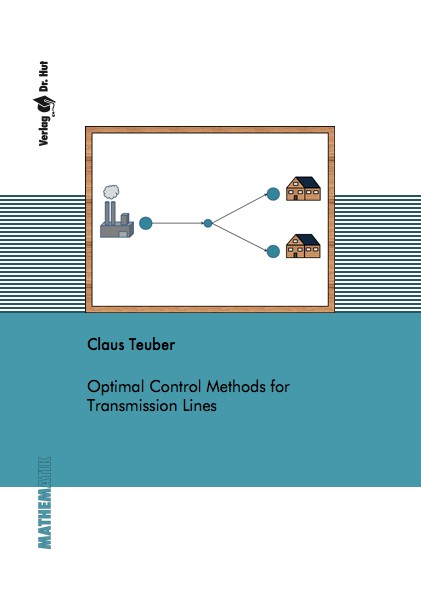Datenbestand vom 15. Oktober 2021aktualisiert am 15. Oktober 2021

# ISBN 978-3-8439-3333-972,00 € inkl. MwSt, zzgl. Versand

978-3-8439-3333-9, Reihe Mathematik

Claus Teuber
Optimal Control Methods for Transmission Lines

140 Seiten, Dissertation Universität Mannheim (2017), Softcover, A5

## Zusammenfassung / Abstract

In this work we examine the mathematical modeling of power networks, their simulation and the optimization under different aspects. Therefore we first derive a well-known model, which is based on the telegraph equations. Since there is no analytical solution available for these equations, we apply numerical schemes and analyze the effect of different model parameters via simulations.

Nevertheless, the focus of this work lies on solving different optimization problems and making use of their structure. In order to do so, we always choose a first discretize-then optimize approach. In a first step we assume that the consumption of all customers in a power network is given for a time interval and compute the optimal production rates of the energy sources or power plants. This implies solving an optimization problem with a quadratic objective function and linear constraints that are due to the discretization of the partial derivatives. To solve this problem we derive a so-called space mapping method, which benefits from a hierarchy of two models of the given problem with different accuracy. We compare this method to established solution methods.

Afterwards we expand the optimization problem by the opportunity of removing single arcs or whole subgrids for a period of time in order to make the transport even more efficient. Therefore we consider two different modeling ideas. These extensions lead to a mixed-integer nonlinear optimization problem, which is in general much harder to solve. For this purpose we introduce a 3-step heuristic based on a known 2-step heuristic from literature. This method is known under the term outer convexification. Moreover we present a second heuristic and apply both heuristics besides established solution methods. Finally, we compare the arising results.